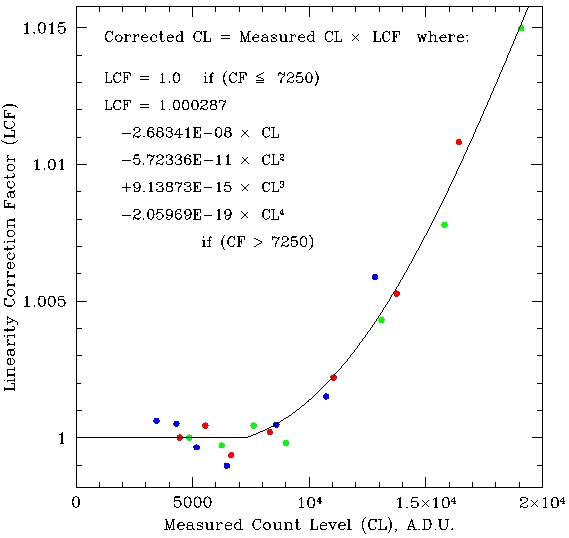# SofI Detector Non-Linearity Correction

The SofI detector is linear up to about 5000 A.D.U. at level of 0.1%. For higher illumination the non-linearity hits in and it reaches about 1% at 13000-14000 A.D.U.

Nota Bene: We strongly urge the users to avoid having raw count levels above 10000 A.D.U. (including the sky level). The correction described here has been tested only partially.

The plots bellow shows the deviation from linearity. To correct for this deviation one has to multiply the raw count level (designated with CL) by the linearity correction factor (designated as LCF) defined by the polynomials given there. The coefficients are intentionally not rounded up to minimize round up errors. These corrections are averaged over the pixels in the central part of the detector.

May 14, 2007 correction:

The raw data for this correction are available here.LCF = 0.9999308 (+/-5.491569E-04)
+9.842033E-09 (+/-3.976830E-07) x CL
-1.240610E-11 (+/-7.976254E-11) x CL^2
+6.953866E-15 (+/-5.842071E-15) x CL^3
-1.908840E-19 (+/-1.403206E-19) x CL^4

July 28, 2010 correction:

The raw data for this correction are available here. Note that the files are trimmed to include only the illuminated part of the detector, to save disk space. The linearity test was run trhree times.LCF = 1.000287 (+/-1.737708E-04)
-2.683405E-08 (+/-5.946259E-08) x CL
-5.723360E-11 (+/-2.185099E-11) x CL^2
+9.138726E-15 (+/-2.391491E-15) x CL^3
-2.059690E-19 (+/-7.501134E-20) x CL^4

May 11, 2012 correction::

The raw data for this correction are available here. Note that the files are trimmed to include only the illuminated part of the detector, to save disk space.LC F = 1.004653 (+/-0.001822944)
-1.306721E-6 (+/-2.773069E-7) x CL
+9.124694E-11 (+/-9.470045E-12) x CL^2

Sept 30, 2012 correction:

The raw data for this correction are available here. Note that the files are trimmed to include only the illuminated part of the detector, to save disk space.LC F = 1.004653 (+/-0.001822944)
-1.306721E-6 (+/-2.773069E-7) x CL
+9.124694E-11 (+/-9.470045E-12) x CL^2